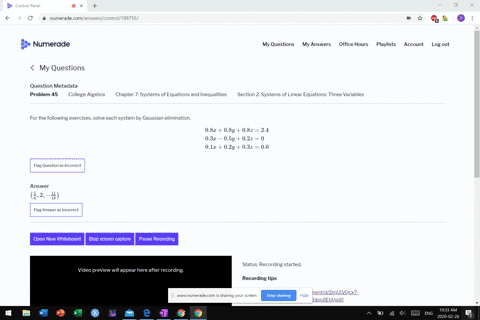Enroll in one of our FREE online STEM bootcamps. Join today and start acing your classes!View Bootcamps03:21
MB
Problem 44

# For the following exercises, solve each system by Gaussian elimination.$$\begin{array}{l}{0.3 x+0.3 y+0.5 z=0.6} \\ {0.4 x+0.4 y+0.4 z=1.8} \\ {0.4 x+0.2 y+0.1 z=1.6}\end{array}$$

## Discussion

You must be signed in to discuss.

## Video Transcript

All right. Question. Number 44 3 Very rose. Three equations don't see an element nation with a bunch of decibels in it. Um, let's start out by getting rid of these decimals in the problem. Okay. Um, single pie. Uh, it's most by all the equations by 10. Okay, so this becomes three acts. Bust three. Why was five z eagle six? This becomes four acts was for why, plus for Z People's 8 10 And this becomes five X was, too. Why, plus the equals 16. All right, now, once we have that, we didn't get a variable to be the same for each one. Um, look like there's anything that that's that's that convenient for any of them. So you see 345 while going to 60 rights will change all of these in the sixties. Right? So more by this equation by money. Okay, It's like issue six. Yes, 60 y watch us. 100 z equals 1 20 Uh, next one, huh? Remote by this one by 15. So I get 60 X plus 60. Why, um, plus 60 z get this, uh, equals. You see, his tux, too meant this, right? Um, 15 times 18 means 270. Okay. And the last one. Times 12. 60 errands. 24. Why? Plus 12 c matty or 12 times 16 which is 192. Right. So these two equations, I'm gonna subtract these equations. I must attract them. I guess I subject down. So 60 minus 60 is 0 61 60 0 100 minus is 40. So I get 40. Easy equals when you see 1 20 minus 2 70 Some is negative. 1 50 All right, so, um, so far, so good. It's like I could just solve this frizzy A second. Uh, this equation here, I and most attract bees. I guess I'll subtract thes down deposit members for that. So 69 60 0 since March 24. 36. Why are you sick? Why 60? Minus 12. 48 plus 48 z equals to 70 miles. 192. All right. Um, let me see. It's This is basically saying that XE is negative. 1 50 divided by 40 which is mega 3.75 Negative. Read 175 I could not take that value. Put it in here and order five watt plus 48 times. They 3.75 Well, 78. All right, most by this out here. So our d a times negative. B 0.75 equals negative. 1 80 appointments. 1 80 people said eight and 1 80 to both sides. 36. Why the walls? 200 and 58 and then 2 58 divided by 36. News 7.16 Repeat, one sits. Repeat two. Today on Ben. I have all three of those and go back to U T. And my equations. I want you find X. And I guess I'm gonna go to this equation year, Have the simplest numbers to work with. So I get three eggs plus three, 7.16 worse bye times. Negative. 3.75 people. Six. This is three X plus. You see the turns 76 this is 21.5 and there five times negative. 3.75 used 18.75. Right. So then move this other stuff. The other side six minus 21. Us. Um, people's 3.2 bucks. 3.2. About three. Can we get one point 18 approximate. Okay, So, um right. Our answer Eyes mortar triple 1.8 for X. You got seven points when 17 were Why? Approximately and then Z isn't all right.